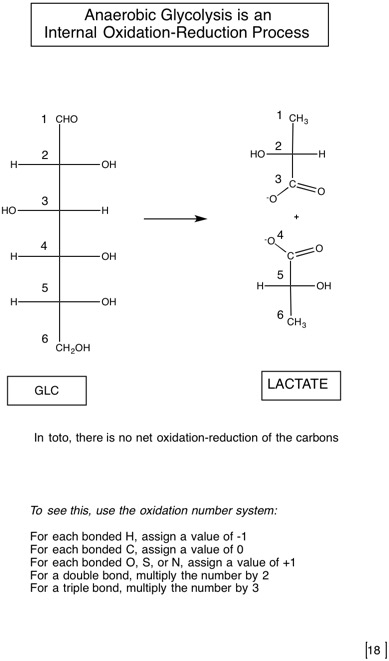# 2.2: Catabolism of glucose-glycolysis as the first pathway tells us about some general metabolic principles

$$\newcommand{\vecs}{\overset { \rightharpoonup} {\mathbf{#1}} }$$ $$\newcommand{\vecd}{\overset{-\!-\!\rightharpoonup}{\vphantom{a}\smash {#1}}}$$$$\newcommand{\id}{\mathrm{id}}$$ $$\newcommand{\Span}{\mathrm{span}}$$ $$\newcommand{\kernel}{\mathrm{null}\,}$$ $$\newcommand{\range}{\mathrm{range}\,}$$ $$\newcommand{\RealPart}{\mathrm{Re}}$$ $$\newcommand{\ImaginaryPart}{\mathrm{Im}}$$ $$\newcommand{\Argument}{\mathrm{Arg}}$$ $$\newcommand{\norm}{\| #1 \|}$$ $$\newcommand{\inner}{\langle #1, #2 \rangle}$$ $$\newcommand{\Span}{\mathrm{span}}$$ $$\newcommand{\id}{\mathrm{id}}$$ $$\newcommand{\Span}{\mathrm{span}}$$ $$\newcommand{\kernel}{\mathrm{null}\,}$$ $$\newcommand{\range}{\mathrm{range}\,}$$ $$\newcommand{\RealPart}{\mathrm{Re}}$$ $$\newcommand{\ImaginaryPart}{\mathrm{Im}}$$ $$\newcommand{\Argument}{\mathrm{Arg}}$$ $$\newcommand{\norm}{\| #1 \|}$$ $$\newcommand{\inner}{\langle #1, #2 \rangle}$$ $$\newcommand{\Span}{\mathrm{span}}$$$$\newcommand{\AA}{\unicode[.8,0]{x212B}}$$Tables of standard free energies of hydrolysis

Table A. Compounds considered to be "High Energy" Compounds

Pi= inorganic phosphate (doesn’t specify a species) = major species at biological pH is $$\mathrm{HPO_{4}^{2-}}$$

Compound

(hydrolysis reaction is shown where more than one is possible)

$$\mathrm{\Delta G^{\circ'}\: (kJ/mol)}$$ Transfer Potential=$$\mathrm{|\Delta G ^{\circ'}|}$$ Compound type

Reason for large $$\mathrm{\Delta G^{\circ'}}$$ of hydrolysis

S=Substrate

P=Product

PEP -61.9 61.9 Enolic phosphate

P tatuomerizes (pyruvate)

P resonance stability (Pi)

1,3-bisPGA -49.3 49.3 Acyl phosphate (phosphoric acid + carboxylic acid)

P ionizaiton (PGA)

Increased P resonance stability (Pi and PGA)

Phosphocreatine -43.0 43.0 Guanidine phoshpate P resonance stability
$$\mathrm{ATP \rightarrow AMP + PPi}$$ -45.6 45.6 Phosphoric acid anhydride

P resonance stability

P ionization

S bond strain due to electrostatic repulsion

$$\mathrm{ADP \rightarrow AMP + Pi}$$ -32.8 32.8 Phosphoric acid anhydirde Same as for $$\mathrm{ATP \rightarrow AMP + P}$$
$$\mathrm{ATP \rightarrow ADP + Pi}$$ -30.5 30.5 Phosphoric acid anhydride Same as for $$\mathrm{ATP \rightarrow AMP + P}$$
UDP-Glucose -31.9 31.9 Sugar nucleotide

P resonance stability

P ionization

Acetyl Coenzyme A -31.4 31.4 Thioester

S no resonance stabilization

P ionization

P resonance stabilization

Sulfur more stable in P

P less charge repulsion

Table B. Compounds considered NOT to be "High Energy" Compounds

 Compound $$\mathrm{\Delta G ^{\circ'}\: (kJ/mol)}$$ Transfer Potential Compound type Reason for $$\mathrm{\Delta G^{\circ'}}$$ of hydrolysis smaller than compounds in Table A. $$\mathrm{AMP \rightarrow adenosine + Pi}$$ -14.2 14.2 Phosphate ester S no destabilizing electrostatic repulsion P (Adenine) doesn't ionize $$\mathrm{Glc-6-P \rightarrow Glc + HPO_{4}^{2-}}$$ -13.8 13.8 Phosphate ester S no electrostatic repulsion

"high energy" bonds are those whose hydrolysis proceeds with $$\mathrm{\Delta G ^{\circ’}}$$ more negative than -25kJ/mol

Most values from Principles of Biochemistry pp. 521.Systematic Common
ATP:hexose 6-phosphotransferase Hexokinase
ATP:GLC 6-phosphotransferase Glucokinase (liver)
GLC-6-P ketolisomerase Phosphoglucose isomerase
ATP:F-6-P 1-phosphotransferase Phosphofructokinase-1 (PFK-1)
F-1,6-bisP G-3-P-lyase aldolase
G-3-P ketolisomerase Triose phosphate isomerase
ATP:3-PGA 1-phosphotransferase Phosphoglycerate kinase
3-PGA 2,3-phosphoisomerase Phosphoglycerate mutase
2-PGA hydro-lyase Enolase
ATP:enol-pyruvate phosphotransferase Pyruvate kinase
Lactate:$$\mathrm{NAD^{+}}$$ oxidoreductase Lactate dehydrogenase
Pyruvate carboxy-lyase Pyruvate decarboxylase
Ethanol:$$\mathrm{NAD^{+}}$$ oxidoreductase Alcohol dehydrogenase2.2: Catabolism of glucose-glycolysis as the first pathway tells us about some general metabolic principles is shared under a not declared license and was authored, remixed, and/or curated by LibreTexts.